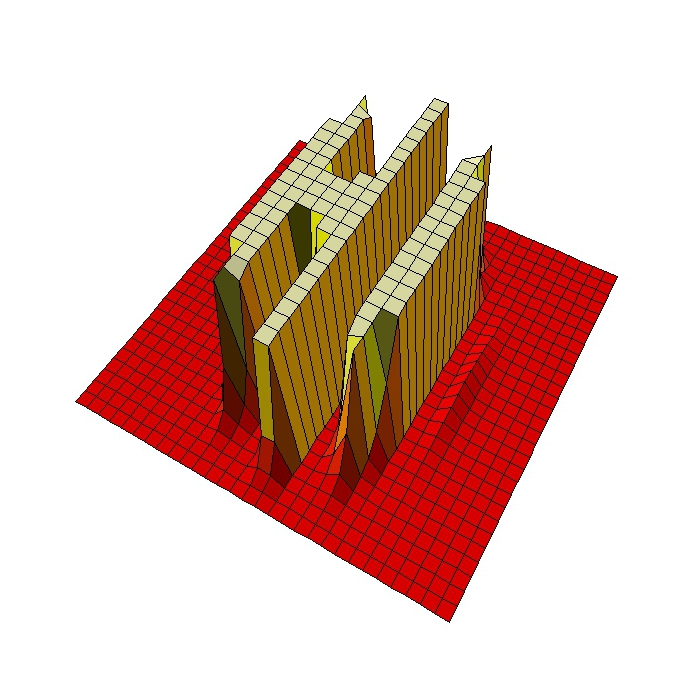# Gummy Worms

Just keep swimming (Dory in Finding Nemo)

Inspired by this post, I decided to create gummy worms like this:Or these:

When I was young I used to eat them.

Do you want to try? This is the code:

```library(rgl)
library(RColorBrewer)
t=seq(1, 6, by=.04)
f = function(a, b, c, d, e, f, t) exp(-a*t)*sin(t*b+c)+exp(-d*t)*sin(t*e+f)
v1=runif(6,0,1e-02)
v2=runif(6, 2, 3)
v3=runif(6,-pi/2,pi/2)
open3d()
spheres3d(x=f(v1, v2, v3, v1, v2, v3, t),
y=f(v1, v2, v3, v1, v2, v3, t),
z=f(v1, v2, v3, v1, v2, v3, t),
```

# Hi

Why do some mathematicians wear a white coat? Are they afraid to be splashed by an integral? (Read on Twitter)

If you run into someone wearing a white coat who tells you something like

e raised to minus 3 by zero point five plus x squared plus y squared between two plus e raised to minus x squared minus y squared between two by cosine of four by x

do not be afraid: is just a harmless mathematician waving to you. Look at this:This is the code to draw these mathematical greetings:

```levelpersp=function(x, y, z, colors=heat.colors, ...) {
## getting the value of the midpoint
zz=(z[-1,-1] + z[-1,-ncol(z)] + z[-nrow(z),-1] + z[-nrow(z),-ncol(z)])/4
## calculating the breaks
breaks=hist(zz, plot=FALSE)\$breaks
## cutting up zz
cols=colors(length(breaks)-1)
zzz=cut(zz, breaks=breaks, labels=cols)
## plotting
persp(x, y, z, col=as.character(zzz), ...)
}
x=seq(-5, 5, length= 30);y=x
f=function(x,y) {exp(-3*((0.5+x)^2+y^2/2))+exp(-x^2-y^2/2)*cos(4*x)}
z=outer(x, y, f)
z[z>.001]=.001;z[z<0]=z[1,1]
levelpersp(x, y, z, theta = 30, phi = 55, expand = 0.5, axes=FALSE, box=FALSE, shade=.25)
```

# 3D-Harmonographs In Motion

I would be delighted to co write a post (Andrew Wyer)

One of the best things about writing a blog is that occasionally you get to know very interesting people. Last October 13th I published this post about the harmonograph, a machine driven by pendulums which creates amazing curves. Two days later someone called Andrew Wyer made this comment about the post:

Hi, I was fascinated by the harmonographs – I remember seeing similar things done on paper on kids tv in the seventies. I took your code and extended it into 3d so I could experiment with the rgl package. I created some beautiful figures (which I would attach if this would let me). In lieu of that here is the code:

I ran his code and I was instantly fascinated: resulting curves were really beautiful. I suggested that we co-write a post and he was delighted with the idea. He proposed to me the following improvement of his own code:

I will try to create an animated gif of one figure

Such a good idea! And no sooner said than done: Andrew rewrote his own code to create stunning animated images of 3D-Harmonograph curves like these:• Andrew creates 3D curves by adding a third oscillation z generated in the same way as x and y and adds a little colour by setting the colour of each point to a colour in the RGB scale related to its point in 3d space
• Function `spheres3d `to produce an interactive plot that you can drag around to view from different angles; function `spin3d `will rotate the figure around the z axis and at 5 rpm in this case and function `movie3d `renders each frame in a temporary png file and then calls ImageMagick to stitch them into an animated gif file. It is necessary to install ImageMagick separately to create the movie.
• Giving it a duration of 12 seconds at 5 rpm is one rotation which at 12 frames per second results in 144 individual png files but these (by default) are temporary and deleted when the gif is produced.

Although I don’t know Andrew personally, I know he is a good partner to work with. Thanks a lot for sharing this work of art with me and allowing me to share it in Ripples as well.

Here you have the code. I like to imagine these curves as orbits of unexplored planets in a galaxy far, far away …

```library(rgl)
library(scatterplot3d)
#Extending the harmonograph into 3d
#Antonio's functions creating the oscillations
xt = function(t) exp(-d1*t)*sin(t*f1+p1)+exp(-d2*t)*sin(t*f2+p2)
yt = function(t) exp(-d3*t)*sin(t*f3+p3)+exp(-d4*t)*sin(t*f4+p4)
#Plus one more
zt = function(t) exp(-d5*t)*sin(t*f5+p5)+exp(-d6*t)*sin(t*f6+p6)
#Sequence to plot over
t=seq(1, 100, by=.001)
#generate some random inputs
f1=jitter(sample(c(2,3),1))
f2=jitter(sample(c(2,3),1))
f3=jitter(sample(c(2,3),1))
f4=jitter(sample(c(2,3),1))
f5=jitter(sample(c(2,3),1))
f6=jitter(sample(c(2,3),1))
d1=runif(1,0,1e-02)
d2=runif(1,0,1e-02)
d3=runif(1,0,1e-02)
d4=runif(1,0,1e-02)
d5=runif(1,0,1e-02)
d6=runif(1,0,1e-02)
p1=runif(1,0,pi)
p2=runif(1,0,pi)
p3=runif(1,0,pi)
p4=runif(1,0,pi)
p5=runif(1,0,pi)
p6=runif(1,0,pi)
#and turn them into oscillations
x = xt(t)
y = yt(t)
z = zt(t)
#create values for colours normalised and related to x,y,z coordinates
cr = abs(z)/max(abs(z))
cg = abs(x)/max(abs(x))
cb = abs(y)/max(abs(y))
dat=data.frame(t, x, y, z, cr, cg ,cb)
#plot the black and white version
with(dat, scatterplot3d(x,y,z, pch=16,cex.symbols=0.25, axis=FALSE ))
with(dat, scatterplot3d(x,y,z, pch=16, color=rgb(cr,cg,cb),cex.symbols=0.25, axis=FALSE ))
#Set the stage for 3d plots
# clear scene:
clear3d("all")
# white background
bg3d(color="white")
#lights...camera...
light3d()
#action
# draw shperes in an rgl window